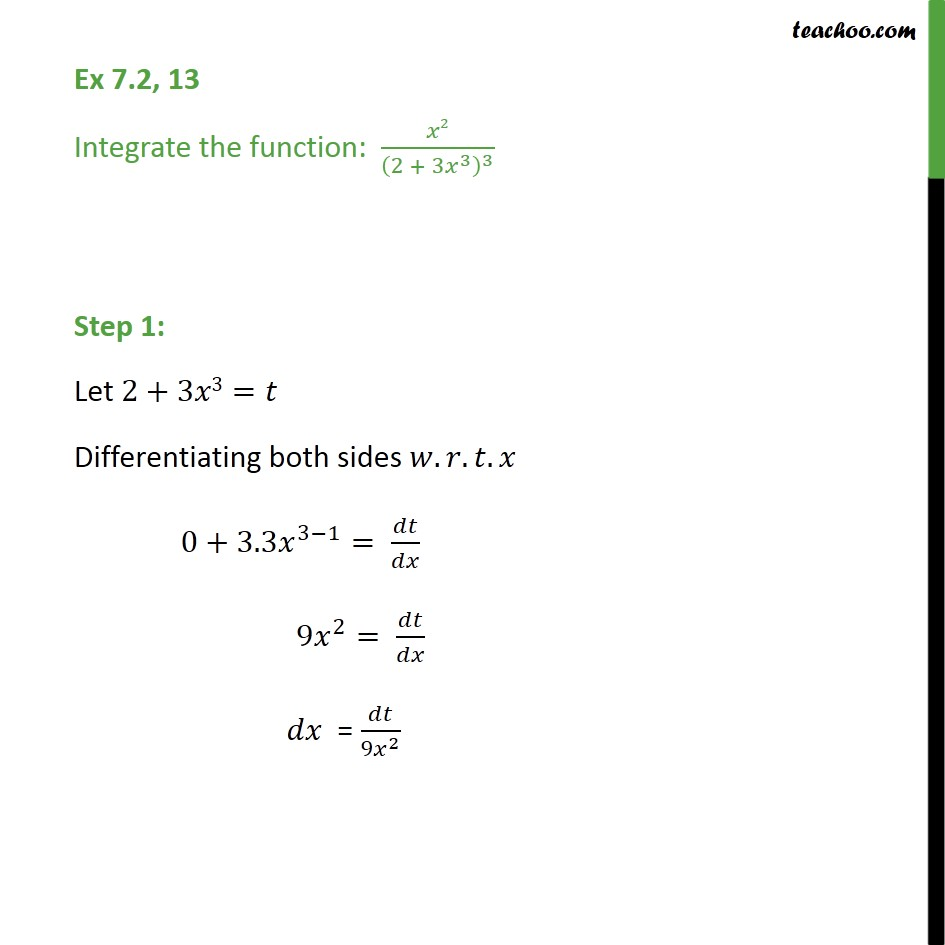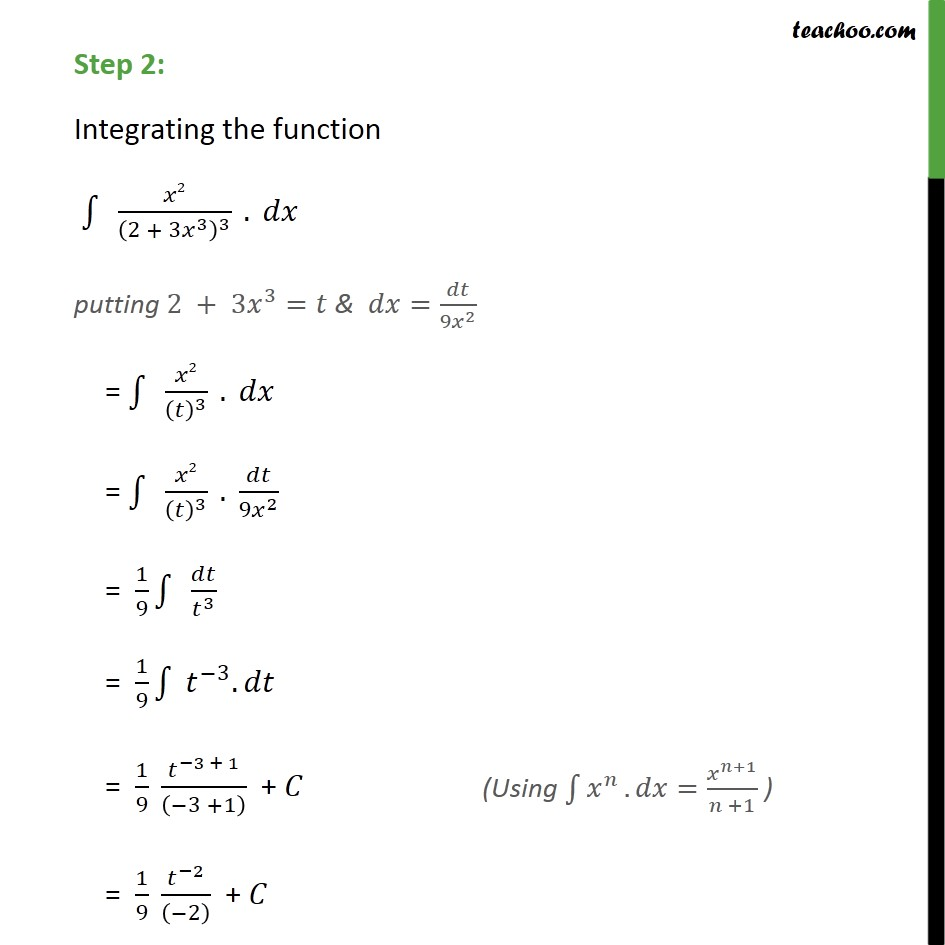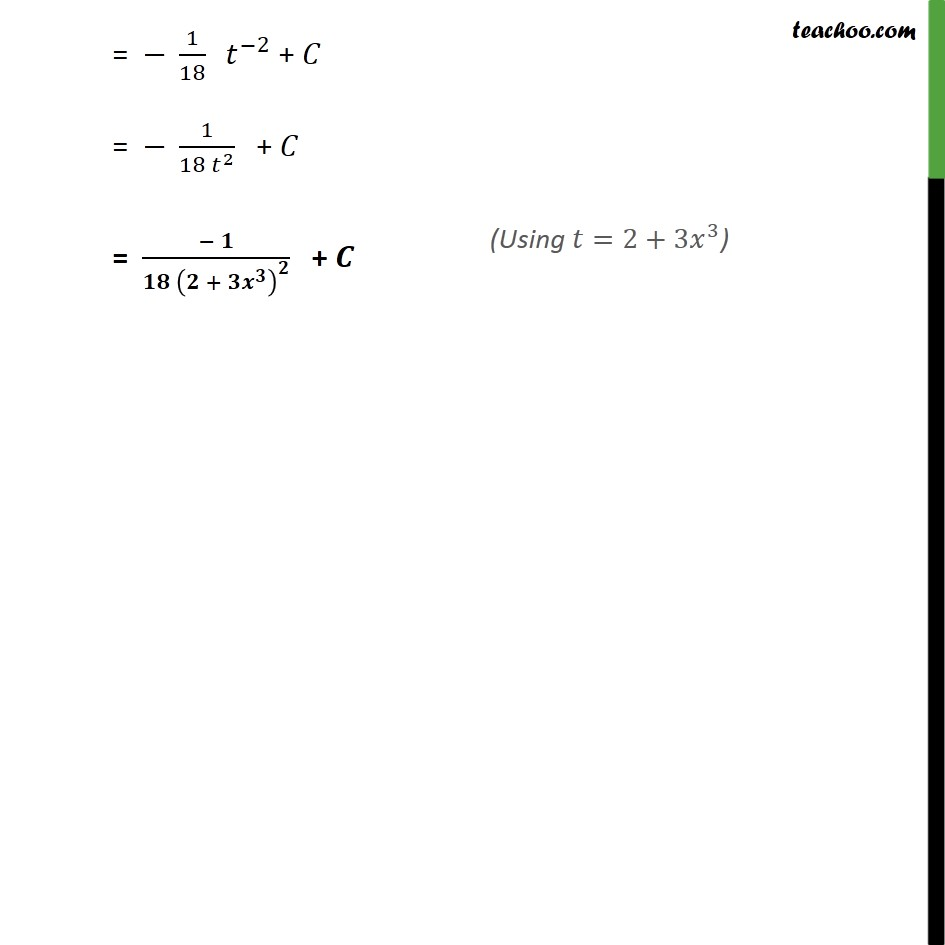Ex 7.2

Chapter 7 Class 12 Integrals
Serial order wiseSolve all your doubts with Teachoo Black (new monthly pack available now!)

### Transcript

Ex 7.2, 13 Integrate the function: 2 2 + 3 3 3 Step 1: Let 2+3 3= Differentiating both sides . . . 0+3.3 3 1 = 9 2 = = 9 2 Step 2: Integrating the function 2 2 + 3 3 3 . putting 2 + 3 3 = & = 9 2 = 2 3 . = 2 3 . 9 2 = 1 9 3 = 1 9 3 . = 1 9 3 + 1 3 +1 + = 1 9 2 2 + = 1 18 2 + = 1 18 2 + = + +